# Tutorials and Fractal Flames

I have finally bit the bullet and started writing tutorials for Visions Of Chaos. Writing good documentation and tutorials is not easy. You need take that step back and try to make all the mathematical variables etc (that are rife in fractals and chaos) simple enough for someone who is not knowledgable about the theories to be able to use the software. I have always tried to make Visions Of Chaos easy enough for beginners to use and advanced enough for nerds to use. Not always an easy balance. I find it much easier to read mathematical research papers than try and explain maths to laymen.

The latest tutorial topic I covered was the fractal flames. In the process I updated and rewrote the code behind the flames to cover the newer variations and implement the original Scott Draves rendering algorithms that all the usual fractal flame rendering apps use. Here are a few sample images and movies using the new code;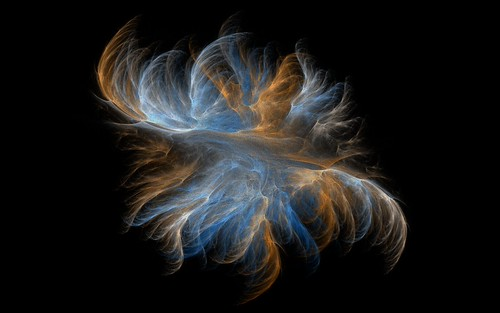One more item off my endless Visions Of Chaos “to do” list implemented.

Jason.

# Further adventures with 3D Strange Attractors

After the original new 3D Strange Attractor formulas I found here I have been playing with alternate formulas and came up with these 5 new types.

Here are the formulas used and some sample images of each type. The a3da[] values are parameters between -1 and +1 that control the shapes.

Rampe 6
xnew=z*sin(a3da*x)-cos(a3da*y)
ynew=x*sin(a3da*y)+cos(a3da*z)
znew=y*sin(a3da*z)-cos(a3da*x)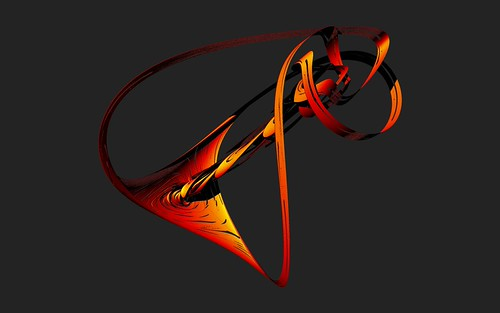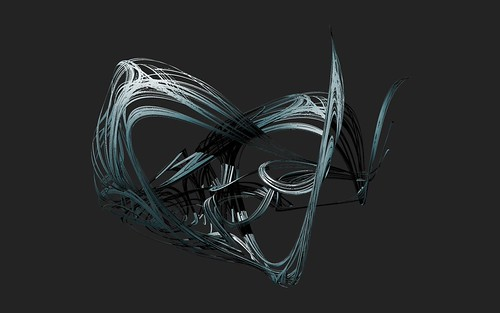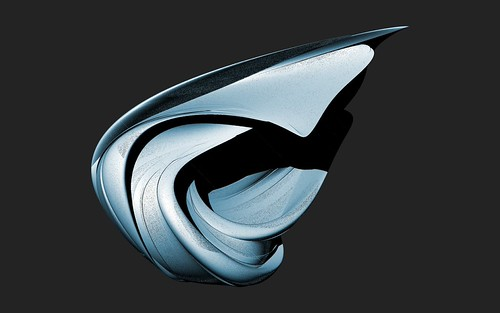Rampe 7
xnew=z*sin(a3da*x)-cos(a3da*y)
ynew=x*cos(a3da*y)+sin(a3da*z)
znew=y*sin(a3da*z)-cos(a3da*x)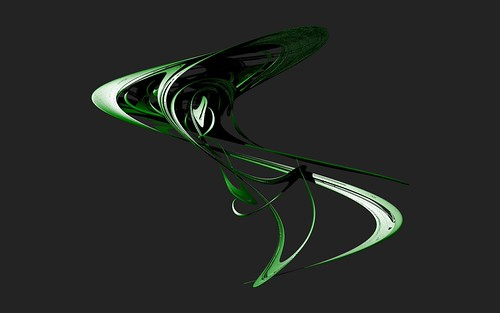Rampe 8
xnew=z*sin(a3da*x)-cos(y)
ynew=x*cos(a3da*y)+sin(z)
znew=y*sin(a3da*z)-cos(x)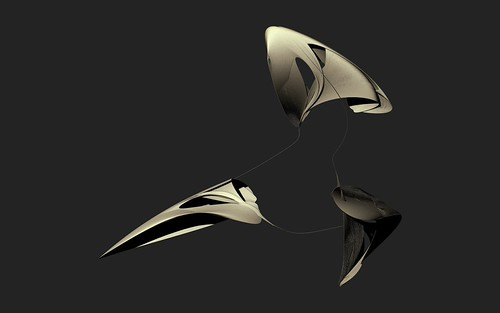Rampe 9
xnew=y*sin(a3da*x)-arccos(a3da*y)+sin(a3da*z)
ynew=z*sin(a3da*x)-arccos(a3da*y)+sin(a3da*z)
znew=x*sin(a3da*x)-arccos(a3da*y)+sin(a3da*z)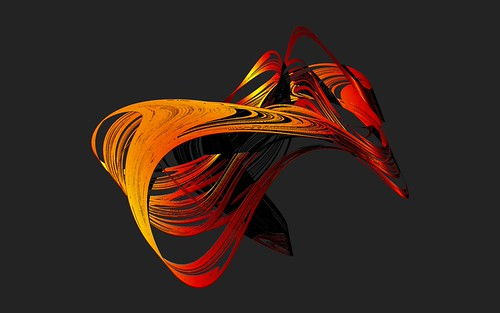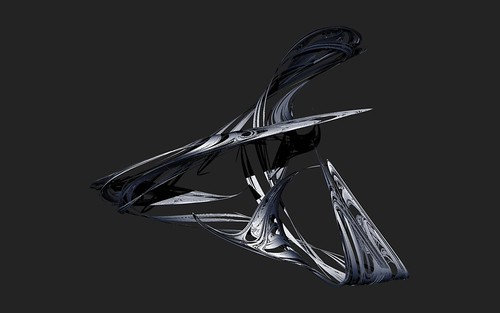Rampe 10
xnew=y*sin(a3da*x)-cos(a3da*y)+arcsin(a3da*z)
ynew=z*sin(a3da*x)-cos(a3da*y)+sin(a3da*z)
znew=x*sin(a3da*x)-cos(a3da*y)+sin(a3da*z)All of the above formulas are now implemented in the latest version of Visions Of Chaos.

I have more ideas to expand new attractor formuals in future versions of Visions Of Chaos. Firstly is to get a decent formula compiler implented so the user can try formulas easily by typing in the 3 formulas for xnew, ynew and znew. Once that works the next obvious evolution is to get it to genetically evolve and mutate the formulas like I have done for Genetic Art in the past.

Jason.

# Powers Of Ten

Powers Of Ten is a great short film from the 60’s (created by Charles and Ray Eames) that gives a unique perspective on sizes from the very large to the very minute. Quoting the video info…

Powers of Ten takes us on an adventure in magnitudes. Starting at a picnic by the lakeside in Chicago, this famous film transports us to the outer edges of the universe. Every ten seconds we view the starting point from ten times farther out until our own galaxy is visible only as a speck of light among many others. Returning to Earth with breathtaking speed, we move inward – into the hand of the sleeping picnicker – with ten times more magnification every ten seconds. Our journey ends inside a proton of a carbon atom within a DNA molecule in a white blood cell.

I saw this as a sequence of images in a book years ago, but never got to watch the video until now.

Jason.

# Orbit Traps

In regards to fractals, orbit traps give a new way to render images. As a fractal is created each pixel of the image is calculated by repeating (iterating) a formula over and over. In the case of the Mandelbrot Set the formula is z=z^2+c. As each iteration happens if you follow or plot the path the complex number z takes at each step you can see the “orbit” the point takes.

To see how these orbits behave, open this shader and move your mouse around. The plotted blue lines are the path the iterations for each pixel go through.

The orbit traps themselves are shapes within the complex plane. If the iterating set of points hits this shape then it is “trapped” and the pixel is then colored depending on how close it is to the trap. Not that easy to explain to someone who does not understand the maths involved (and no doubt if you read the above and are not into math then you have a “what the!?” expression now).

Here is the famous Mandelbrot Set.If the “cross” orbit trap is applied you get an image like the following.The main centered white cross shape is the main trap. Any time the orbit of a pixel hits within the cross shape it stops iterating and is shaded based on its location within the trap. If only the trap shape is displayed the result is like this image.There are numerous styles and types of orbit traps out there. Here is another sample of the above Mandelbrot Set rendered with the “stalks” orbit trap.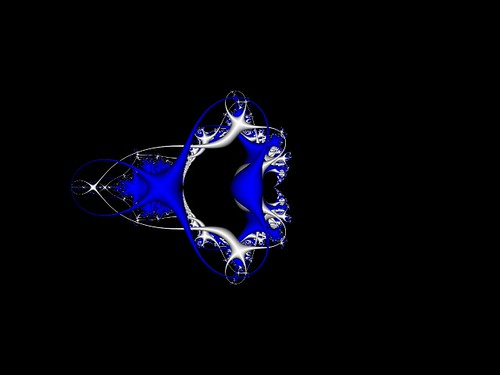When you zoom into these orbit trap images you get some more interesting results.Source Code

The source code to these orbit traps is included in the Custom Formula Editor of Visions of Chaos. Here are the 5 shaders here for people who want to see how they work.

Root-Finding Fractals Orbit Traps

After expanding the root-finding fractals mode in Visions Of Chaos I have now added the ability to render orbit traps within them. The results are as interesting or more interesting than the Mandelbrot orbit trap images. Here are a few example images.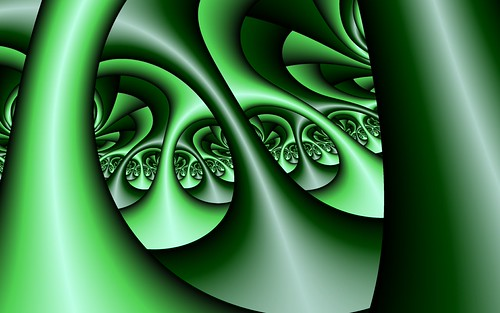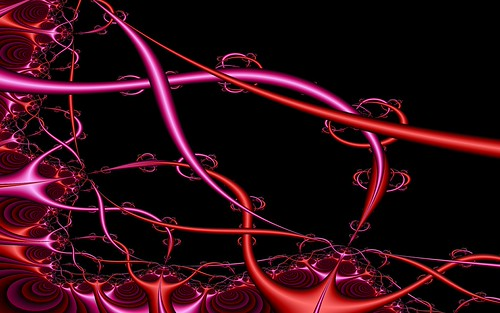Here is a zoom into the above Newton Fractal using the cross orbit trap and shaded with Perlin Noise.

Even though the movie has a semi 3D appearance of going through a forest of fractal tree trunks the frames are pure 2D. It is the orbit traps that give it the 3D look.

There are many more styles of orbit traps to use and I will no doubt be playing and experimenting with new varieties for years to come.